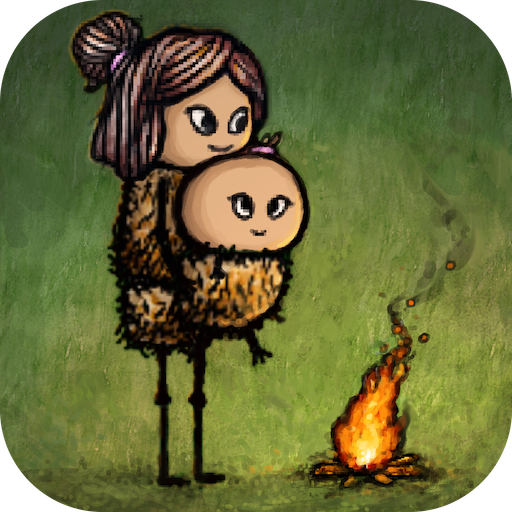# Longer lifespan

Considering it’s not 1 hour 1 life and is an unofficial adaptation I was thinking to maybe start to differ from the standard concept and add age verity. This may include a system that from 60 the odds of you randomly die ing every year increases for example 61 =1/100 62=2/100 63=3/100 64=4/100 65=6/100 66=8/100 67=9/100 68=10/100 69=11/100 70=13/100 71=16/100 72=18/100 73=20/100 74=22/100 75=24/100 76=26/100 77=28/100 78=30/100 79=32/100 80=35/100 81=40/100 82=45/100 83=50/100 84=55/100 85=60/100 86=65/100 87=70/100 88=75/100 89=80/100 90 and up =85/100 then 100=100/100 I think this method would really add some verity to the game so plz try to add it because right now it’s just a mobile copy of 1 hour 1 life somthing like this would make the game match better comment if you agree with me

2 Likes

I mean it kinda defeats the purpose of the fact that you have strictly an hour to do everything that you need to do, but it would be interesting to see a person living for some extra years depending on their overall health (amount of yellow fevers, having low/high temperatures) or yum chain length.

1 Like

아니요, 게임의 목적에 위배됩니다.

공정하게 말하면 Ohol의 목적에 위배되지만 YAH는 자체적으로 할 수 있습니다.